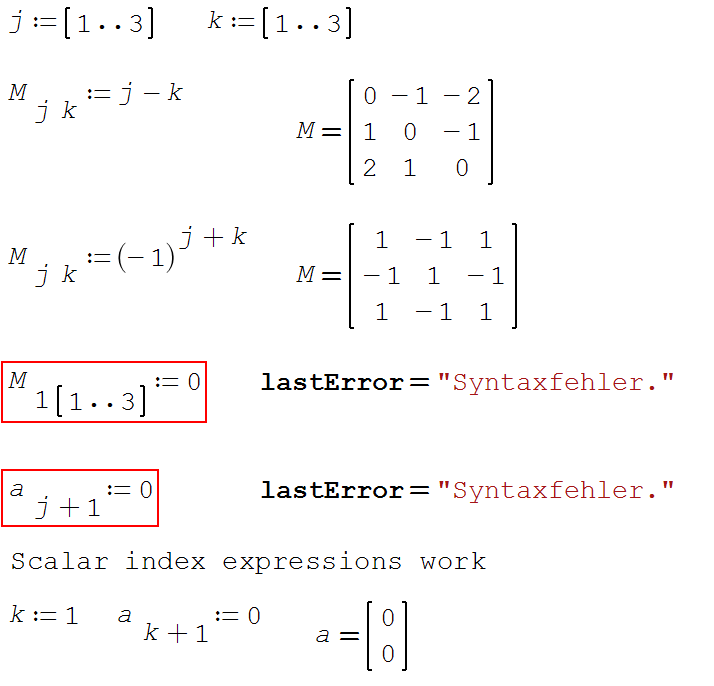Feature SS-3513
1 vote

### Allow index expressions for implicit assignment loops

Created by Martin Kraska on 3/2/2018 1:57 PM Last Updated by Martin Kraska on 3/2/2018 7:18 PM
%
(hrs)
Logged: 0   (hrs)

Description

Ranged indices, be it an expression or a variable, work work on the right hand side for access to existing vector or matrix elements.
This concept can be used for any expression except assignment targets.

If assignemts to vector or matrix elements have to be executed for multiple index values, then you can use explicit for-loops (programming) or implicit loops. These are generated by just using vector-valued variables (general vector-valued expressions aren't allowed) as indices in the assignment target.

I propose to extend the concept of implicit loops to any vector-valued index expressions. A naive implementation concept would be:

If the index of an assignment target is an expression:

Check for defined variables that occure on both right hand side and left hand side. If such variables are found

Generate a loop for each of these variables over the defined values

execute the assignemt by computing the target indices and the rhs expression in each iteration

Otherwise

compute the rhs (which is independent of any variables occuring in the target index expression)

compute the index expression.

Assign rhs value to all elements covered by index expression.Martin Kraska (Friday, March 2, 2018 7:18 PM) #

I am excited! What a pace!  Thank you, This is another change which streamlines the handbook and makes using SMath Studio more straightforward.

BTW, do you see any chance for implementing classical index equations as known from linear algebra? That would require loops and optional/suppressable summation over repeated indexes on right hand side

a[i*b[i would be the scalar product of two vectors a and b

a[i;i would be the trace of a matrix

a[i;j*b[j would be the product of a matrix and a vector

I admit, that this idea is very crude and would require careful specification/discussion of what finally is to be implemented.. But I am a big fan of having SMath close to natural math notation.smath (Friday, March 2, 2018 6:53 PM) #

Thank you!

Implemented.